Function Repository Resource:

# ActuarialCommutation

Creates an Association containing values for the six standard commutation functions used in actuarial mathematics

Contributed by: Seth J. Chandler
 ResourceFunction["ActuarialCommutation"][l,v] computes values for the six standard commutation functions ("D","N","S", "C","M","R") as a function of surviving population fraction l and discount rate v.

## Details and Options

ResourceFunction["ActuarialCommutation"] functions can be used to compute a number of quantities of interest in actuarial mathematics, such as the value of an annuity or death benefit.
The parameter l is a list containing the fraction of the initial population surviving at the start of each time unit.
The parameter v is a discount rate generally between 0 and 1, showing the value of money received one year from now.
The "ReturnForm" option directs the form in which the data is returned: permissible option values are as follows:
"FunctionAssociation" returns an association in which the commutation function names are the keys and the values are the values of each function at each successive unit of time;
"TimeAssociation" returns a nested association in which the outer keys are the successive units of time and the values are associations in which the keys are the names of the commutation functions and the values are the value of that function at the specified unit of time.
"FunctionValues" returns a nested list in which each outer list represents the values of a commutation function at a successive unit of time. The lists are ordered as "D", "N", "S", "C", "M", "R". For example, the fourth element of the returned values contains a list of the values for the "C" commutation function.
“TimeValues” returns a nested list in which each outer list represents a unit of time. Each inner list contains six values, one for each of the six commutation functions and in the order "D", "N", "S", "C", "M", "R".
The "InitialTime" option can (and probably should) be used when the mortality table begins at an age other than 0.
Although commutation functions and tables displaying them are used less frequently than in times when computation was less accessible, they are still a useful tool in swiftly computing quanities of interest in actuarial mathematics.

## Examples

### Basic Examples (3)

Compute the commutation functions for a mortality table that goes from 1 to 0 in five periods and a discount rate of 0.9:

 In:=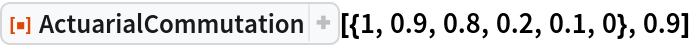Out=Do the same computation as immediately above but return the values as a nested Association in which the outer keys represent years. The result is converted into a Dataset to aid visualization:

 In:=Out=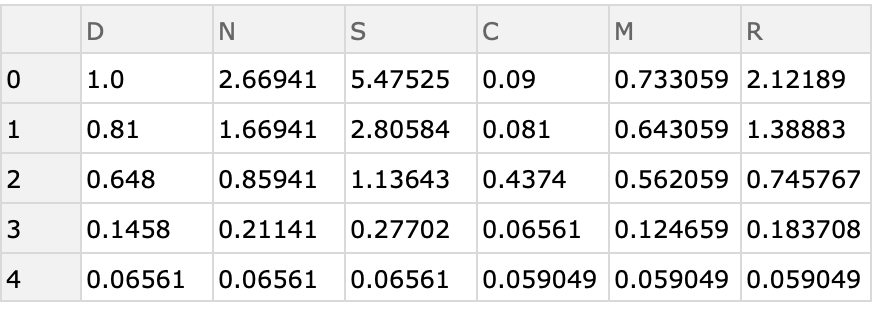Do the same computation as immediately above, but assume the starting age for the mortality vector is age 20:

 In:=Out=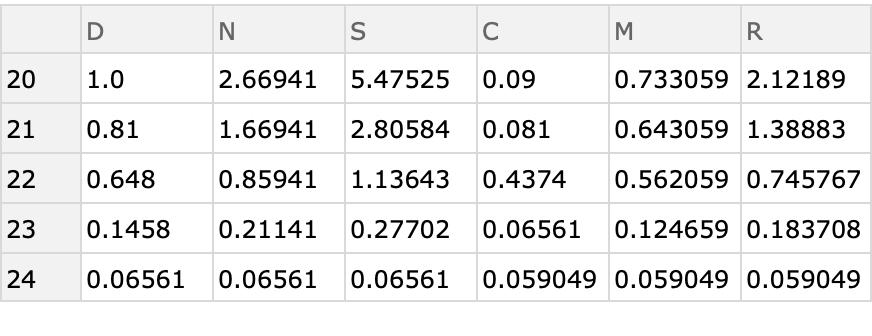### Scope (3)

Exact values will be provided where rational numbers are used for the inputs:

 In:=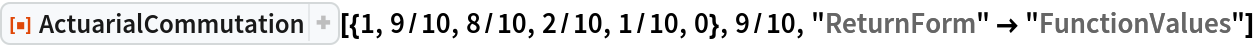Out=The function works on symbolic arguments:

 In:=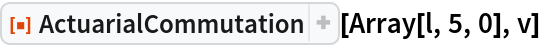Out=By adjusting the discount rate, the function can be used for mortality tables that go by time units other than a year. Compute the commutation functions of a mortality table that goes by 10-year periods when the annual discount rate is 0.95:

 In:=Out=### Options (2)

Changing the "ReturnForm" option changes the form of the output:

 In:=Out=Changing the "InitialTime" option changes the keys of the "TimeAssociation" return form; the values of each association remain the same:

 In:=Out=### Applications (8)

Get the commutation functions for males based on "U.S. Historical Population Mortality Rates 2000-2017 for 2017" and a discount rate of 0.95:

 In:=Out=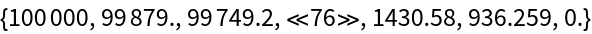In:=Out=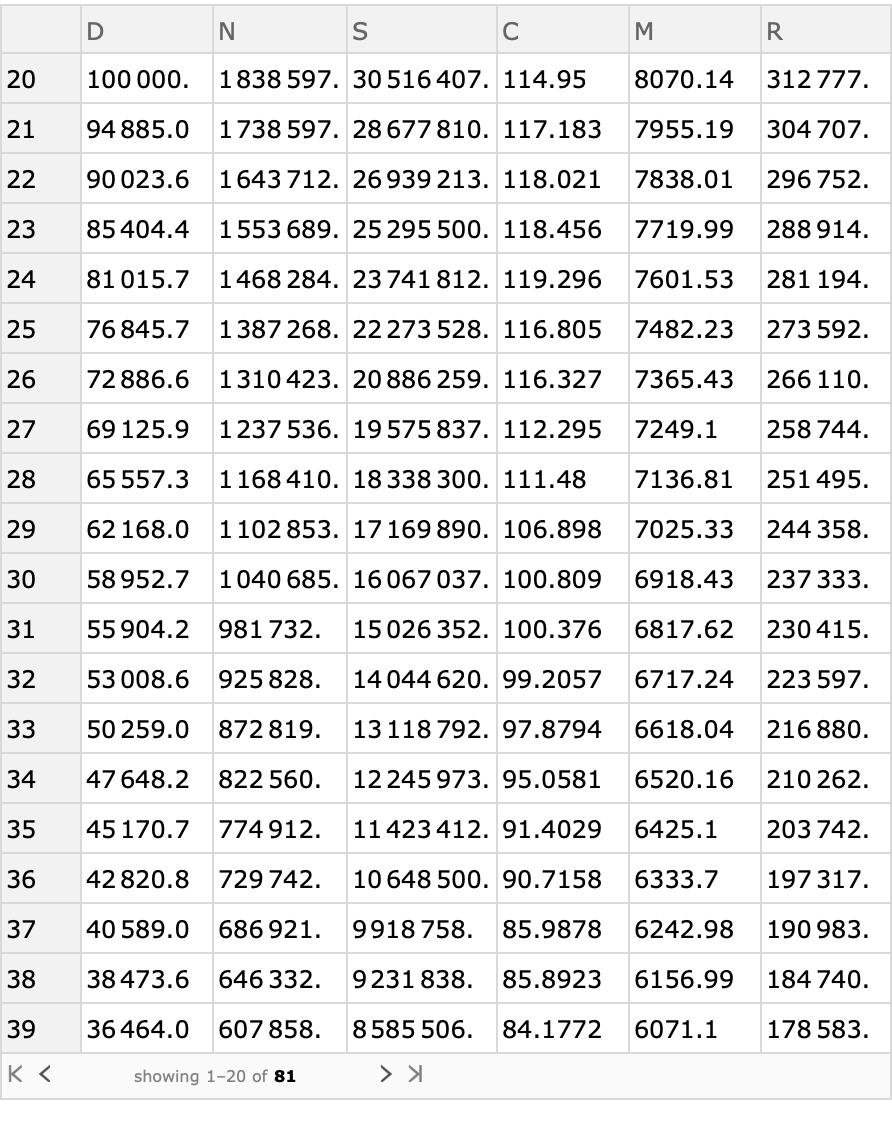Use the commutation dataset to compute the value of an "increasing annuity" issued to a 60 year old male that pays \$1 its first year, \$2 its second year, and one additional dollar each year thereafter:

 In:=Out=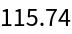For a person aged 33, compute the net single premium for a 20-year term insurance payable at the end of the year of death, where \$1 is paid if the death occurs in the first year, \$2 is paid is the death occurs in the second year, and so on:

 In:=Out=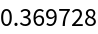Use the commutation dataset to plot the level annual premium for a term life insurance policy of 20 years as a function of the issue age of the insured:

 In:=Out=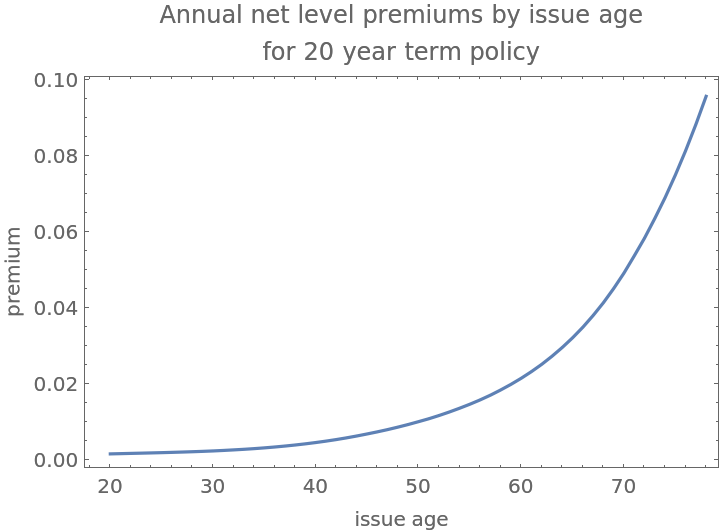Use the commutation dataset to compute the annual level premium (paid at the beginning of the year) per \$1 of whole life coverage for a purchaser with issue age of 25:

 In:=Out=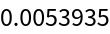Use the same dataset to plot the retrospective reserve on this policy according tothe attained age of the insured:

 In:=Out=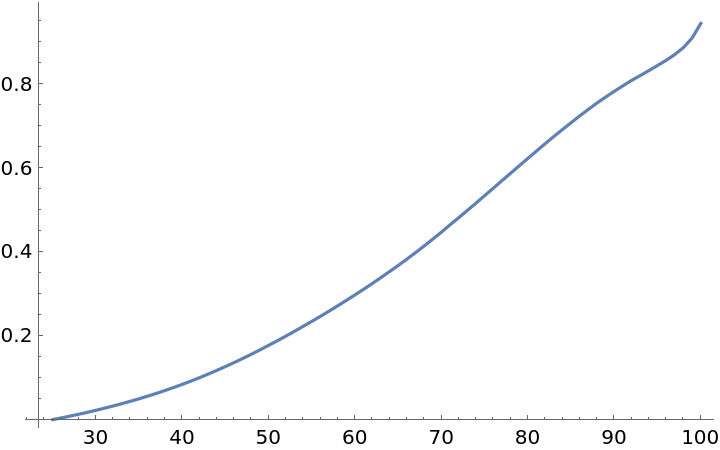Compute the net single premium needed to fund a life insurance policy of \$1,000,000 on a 61 year old male for 10 years, with death benefits paid at the end of the year of death:

 In:=Out=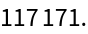Do the same computation using the commutation dataset but make the notation more transparent:

 In:=Out=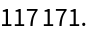### Properties and Relations (1)

The resource function MortalityConvert permits use of mortality data provided as a hazard or probability instead of as survival:

 In:=Out=### Neat Examples (1)

Determine the amount an insurer would be willing to pay to rid itself of a \$1,000,000 insurance policy issued to a 25 year-old priced under the mortality table contained in "U.S. Historical Population Mortality Rates 2000-2017 for 2017" when the insurer becomes aware that the insured’s hazards are 80% greater than contained in the table:

 In:=Out=In:=Out=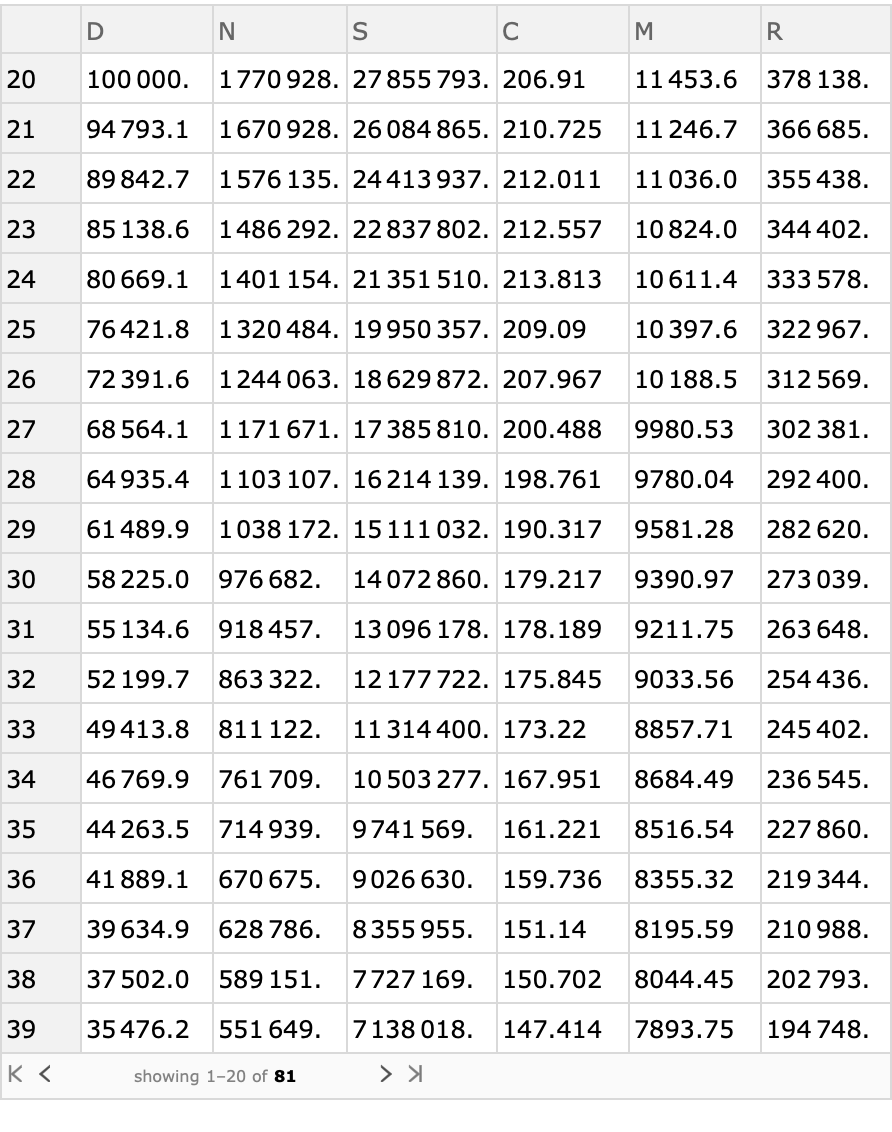In:=Out=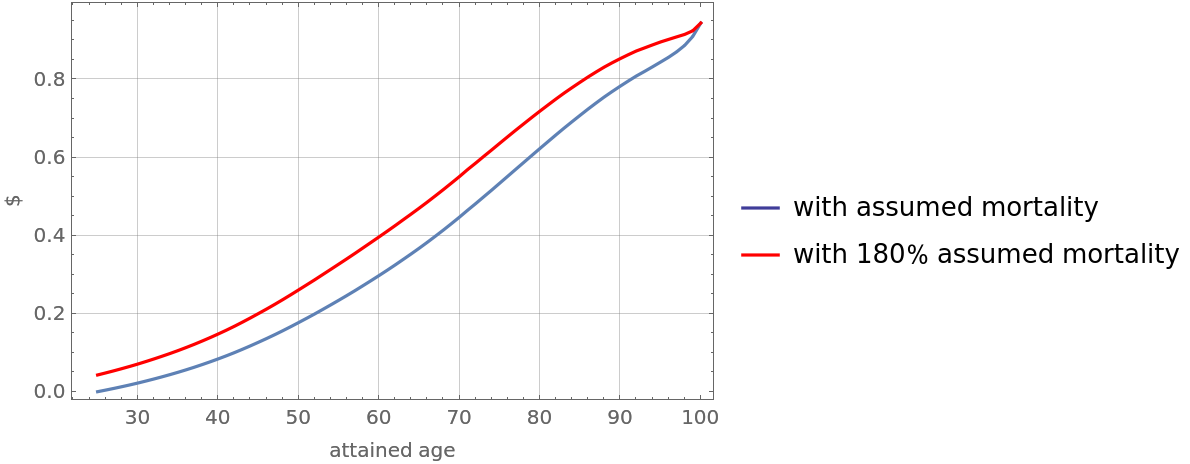Seth J. Chandler

## Version History

• 1.0.0 – 28 August 2019